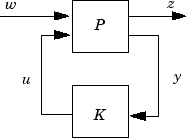# hinfgs

Synthesis of gain-scheduled H controllers

## Syntax

```[gopt,pdK,R,S] = hinfgs(pdP,r,gmin,tol,tolred)
```

## Description

Given an affine parameter-dependent plant

`$P\left\{\begin{array}{c}\stackrel{˙}{x}=A\left(p\right)x+{B}_{1}\left(p\right)w+{B}_{2}u\\ z={C}_{1}\left(p\right)x+{D}_{11}\left(p\right)w+{D}_{12}u\\ y={C}_{2}x+{D}_{21}w+{D}_{22}u\end{array}$`

where the time-varying parameter vector p(t) ranges in a box and is measured in real time, `hinfgs` seeks an affine parameter-dependent controller

`$K\left\{\begin{array}{c}\stackrel{˙}{\zeta }={A}_{K}\left(p\right)\zeta +{B}_{K}\left(p\right)y\\ u={C}_{K}\left(p\right)\zeta +{D}_{K}\left(P\right)y\end{array}$`

scheduled by the measurements of p(t) and such that

• K stabilizes the closed-loop systemfor all admissible parameter trajectories p(t)

• K minimizes the closed-loop quadratic H performance from w to z.

The description `pdP` of the parameter-dependent plant P is specified with `psys` and the vector `r` gives the number of controller inputs and outputs (set `r=[p2,m2]` if yRp2 and uRm2). Note that `hinfgs` also accepts the polytopic model of P returned, e.g., by `aff2pol`.

`hinfgs` returns the optimal closed-loop quadratic performance `gopt` and a polytopic description of the gain-scheduled controller `pdK`. To test if a closed-loop quadratic performance γ is achievable, set the third input `gmin` to γ. The arguments `tol` and `tolred` control the required relative accuracy on `gopt` and the threshold for order reduction. Finally, `hinfgs` also returns solutions R, S of the characteristic LMI system.

## Controller Implementation

The gain-scheduled controller `pdK` is parametrized by p(t) and characterized by the values KΠj of $\left(\begin{array}{cc}{A}_{K}\left(p\right)& {B}_{K}\left(p\right)\\ {C}_{K}\left(p\right)& {D}_{K}\left(p\right)\end{array}\right)$ at the corners ³j of the parameter box. The command

```Kj = psinfo(pdK,'sys',j) ```

returns the j-th vertex controller KΠj while

```pv = psinfo(pdP,'par') vertx = polydec(pv) Pj = vertx(:,j) ```

gives the corresponding corner ³j of the parameter box (`pv` is the parameter vector description).

The controller scheduling should be performed as follows. Given the measurements p(t) of the parameters at time t,

1. Express p(t) as a convex combination of the ³j:

This convex decomposition is computed by `polydec`.

2. Compute the controller state-space matrices at time t as the convex combination of the vertex controllers KΠj:

`$\left(\begin{array}{cc}{A}_{K}\left(t\right)& {B}_{K}\left(t\right)\\ {C}_{K}\left(t\right)& {D}_{K}\left(t\right)\end{array}\right)=\sum _{i=1}^{N}{\alpha }_{j}{K}_{\Pi }{}_{{}_{\iota }}.$`
3. Use AK(t), BK(t), CK(t), DK(t) to update the controller state-space equations.

## References

Apkarian, P., P. Gahinet, and G. Becker, “Self-Scheduled H Control of Linear Parameter-Varying Systems,” Automatica, 31 (1995), pp. 1251–1261.

Becker, G., Packard, P., “Robust Performance of Linear-Parametrically Varying Systems Using Parametrically-Dependent Linear Feedback,” Systems and Control Letters, 23 (1994), pp. 205–215.

Packard, A., “Gain Scheduling via Linear Fractional Transformations,” Syst. Contr. Letters, 22 (1994), pp. 79–92.

## Version History

Introduced before R2006a